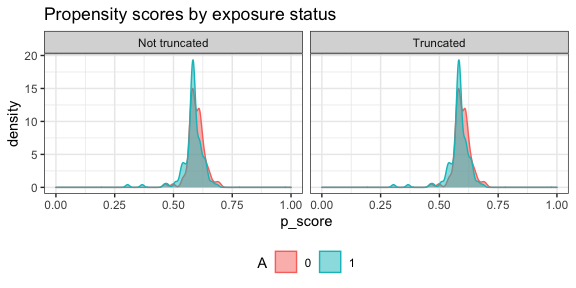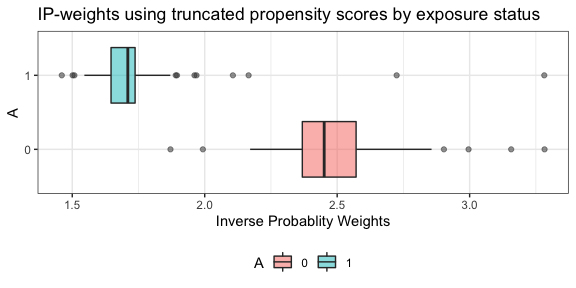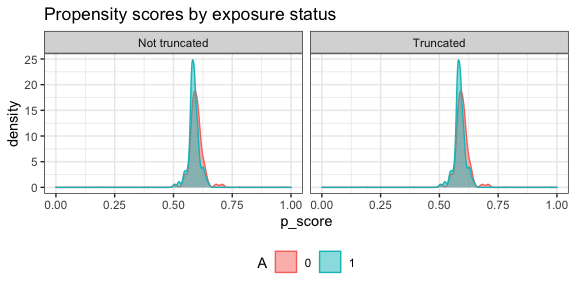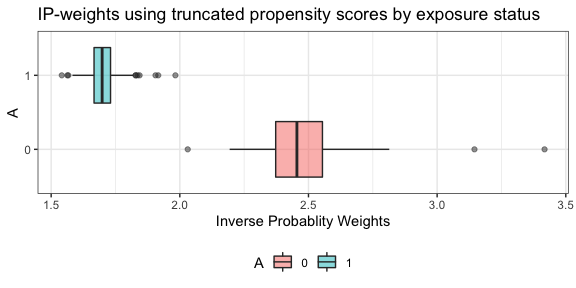# Getting Started with AIPW

Contents:

## Installation

1. Install AIPW from GitHub
install.packages("remotes")
remotes::install_github("yqzhong7/AIPW")

* CRAN version only supports SuperLearner and tmle. Please install the Github version (master branch) to use sl3 and tmle3.

1. Install SuperLearner or sl3
#SuperLearner
install.packages("SuperLearner")
#sl3
remotes::install_github("tlverse/sl3")
install.packages("Rsolnp")

## Input data for analyses

library(AIPW)
library(SuperLearner)
#> Super Learner
#> Version: 2.0-26
#> Package created on 2019-10-27
library(ggplot2)
set.seed(123)
data("eager_sim_obs")
cov = c("eligibility","loss_num","age", "time_try_pregnant","BMI","meanAP")

## Using AIPW to estimate the average treatment effect

### One line version (Method chaining from R6class)

Using native AIPW class allows users to define different covariate sets for the exposure and the outcome models, respectively.

AIPW_SL <- AIPW$new(Y= eager_sim_obs$sim_Y,
A= eager_sim_obs$sim_A, W= subset(eager_sim_obs,select=cov), Q.SL.library = c("SL.mean","SL.glm"), g.SL.library = c("SL.mean","SL.glm"), k_split = 10, verbose=FALSE)$
fit()$#Default truncation is set to 0.025; using 0.25 here is for illustrative purposes and not recommended summary(g.bound = c(0.25,0.75))$
plot.p_score()$plot.ip_weights()### A more detailed tutorial #### 1. Create an AIPW object • ##### Use SuperLearner libraries library(AIPW) library(SuperLearner) #SuperLearner libraries for outcome (Q) and exposure models (g) sl.lib <- c("SL.mean","SL.glm") #construct an aipw object for later estimations AIPW_SL <- AIPW$new(Y= eager_sim_obs$sim_Y, A= eager_sim_obs$sim_A,
W= subset(eager_sim_obs,select=cov),
Q.SL.library = sl.lib,
g.SL.library = sl.lib,
k_split = 10,
verbose=FALSE)

If outcome is missing, analysis assumes missing at random (MAR) by estimating propensity scores with I(A=a, observed=1). Missing exposure is not supported.

#### 2. Fit the AIPW object

This step will fit the data stored in the AIPW object to obtain estimates for later average treatment effect calculations.

#fit the AIPW_SL object
AIPW_SL$fit() # or you can use stratified_fit # AIPW_SL$stratified_fit()

#### 3. Calculate average treatment effects

• ##### Estimate the ATE with propensity scores truncation
#estimate the average causal effects from the fitted AIPW_SL object
AIPW_SL$summary(g.bound = 0.25) #propensity score truncation  • ##### Check the balance of propensity scores and inverse probability weights by exposure status after truncation library(ggplot2) AIPW_SL$plot.p_score()AIPW_SL$plot.ip_weights()#### 4. Calculate average treatment effects among the treated/controls • ##### stratified_fit() fits the outcome model by exposure status while fit() does not. Hence, stratified_fit() must be used to compute ATT/ATC (Kennedy et al. 2015) suppressWarnings({ AIPW_SL$stratified_fit()$summary() }) ## Parallelization with future.apply In default setting, the AIPW$fit() method will be run sequentially. The current version of AIPW package supports parallel processing implemented by future.apply package under the future framework. Before creating a AIPW object, simply use future::plan() to enable parallelization and set.seed() to take care of the random number generation (RNG) problem:

# install.packages("future.apply")
library(future.apply)
plan(multiprocess, workers=2, gc=T)
set.seed(888)
AIPW_SL <- AIPW$new(Y= eager_sim_obs$sim_Y,
A= eager_sim_obs$sim_A, W= subset(eager_sim_obs,select=cov), Q.SL.library = sl3.lib, g.SL.library = sl3.lib, k_split = 10, verbose=FALSE)$fit()$summary() ## Use tmle fitted object as input AIPW shares similar intermediate estimates (nuisance functions) with the Targeted Maximum Likelihood / Minimum Loss-Based Estimation (TMLE). Therefore, AIPW_tmle class is designed for using tmle fitted object as input. Details about these two packages can be found here and here. This feature is designed for debugging and easy comparisons across these three packages because cross-fitting procedures are different in tmle. In addition, this feature does not support ATT outputs. #### tmle As shown in the message, tmle only support cross-fitting in the outcome model. # install.packages("tmle") library(tmle) library(SuperLearner) tmle_fit <- tmle(Y=eager_sim_obs$sim_Y,
A=eager_sim_obs$sim_A, W=eager_sim_obs[,-1:-2], Q.SL.library=c("SL.mean","SL.glm"), g.SL.library=c("SL.mean","SL.glm"), family="binomial", cvQinit = TRUE) cat("\nEstimates from TMLE\n") unlist(tmle_fit$estimates$ATE) unlist(tmle_fit$estimates$RR) unlist(tmle_fit$estimates$OR) cat("\nEstimates from AIPW\n") a_tmle <- AIPW_tmle$
new(A=eager_sim_obs$sim_A,Y=eager_sim_obs$sim_Y,tmle_fit = tmle_fit,verbose = TRUE)\$
summary(g.bound=0.025)